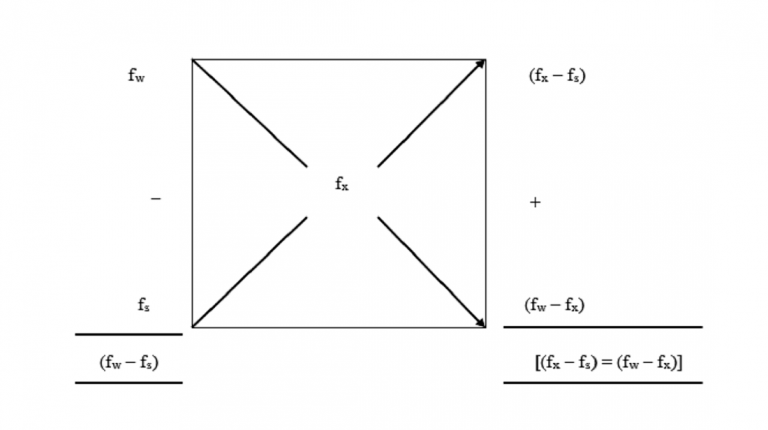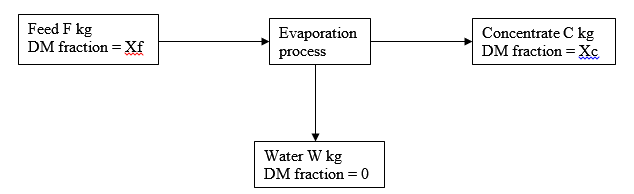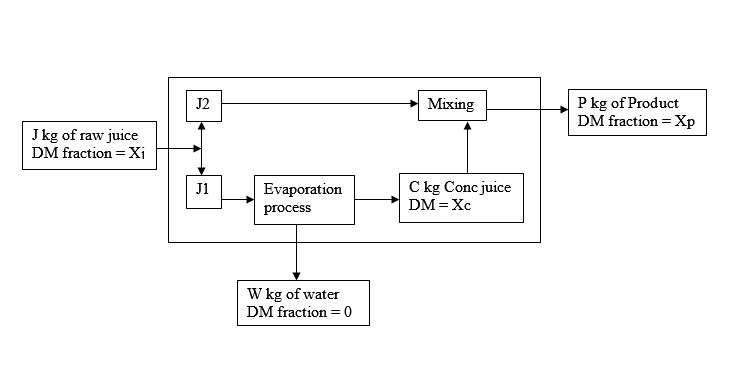0
AgriQuora . 28th Jun, 2021

### Pearson's Square & Mass Balancing Methods of StandardizationIn this post, we shall be discussing two methods of material standardization, namely, Pearson Square method and Mass Balancing method. We'll start with the Pearson Square method and then later on delve into the mass balancing method. Our case studies will be based on dairy and juice processing.

## The Pearson Square Method

Milk standardization is important in the dairy industry because it is used to ensure that every consumer gets milk with constant fat content and consistency. Primarily, milk may be separated into two products; cream with high fat content (about 40% fat) and skimmed milk (about 0.03% fat) using a centrifugal separator.

The process of standardization involves reduction of fat content in milk from the natural minimum of 3.25%. The cream extracted from the standardization process facilitates processing of other dairy products such as ice cream, butter, and ghee.

Milk standardization can take two forms i.e. either partial separation or mixing of skimmed and whole milk. Mass balancing plays a key role in successful standardization of milk.

#### Example 1

2000 kg of milk (with 87.6% water, 3.8% fat, 3.2% protein, 4.6% lactose, and 0.7% ash content) has to be reduced in fat content from 3.8% to 2.5% by removal of cream with 40% fat content from the milk. How much milk will have to be removed?

#### Solution.

TMB: 2000 = C + M

FMB:   2000*0.038 = 0.4*C + 0.025*M

2000*0.025 = 0.025*C + 0.025*M

Solving the equations will give the values of C = 69.3 kg and the remaining milk M = 1930.7 kg.

#### Example 2

If 3000 kg of the same milk used in the previous example is separated into cream with 45% fat and skimmed milk with 0.05% fat, how much cream and skimmed milk are expected assuming no losses?

#### Solution

TMB:   3000 = C + M

FMB:   3000*0.038 = 0.45*C + 0.0.0005*S

Solving the equations will give C = 250.3 kg and S = 2749.7 kg

Consider mixing W kg of whole milk with a fat content of fw and S kg of skimmed milk with a fat content of fs to obtain X kg of standardized milk with fx.

There is only one value, pw, for the fraction of W in X and one value, Ps = (1-Pw) for the fraction of S in X.

The challenge to the processor is to determine these values.

##### Using mass balance:

TMB:   W + S = X, and W + S = W + S

FMB:   Wfw + Sfs = Xfx

Xfx = fx(W + S)

Expanding the equations

Wfw + Sfs = Wfx + Sfx

W(fw – fx) = S(fx – fs)

W/S = (fx – fs)/(fw – fs)

W/(W + S) = (fx – fs)/[(fx – fs) + (fw + fs)] = Pw = W/X

S/(W + S) = (fw – fs)/[(fx – fs) + (fw – fs)] = Ps = S/X

NB: (fx – fs) + (fw – fs) = (fw – fs).

### Milk Standardization Using Pearson’s Square Method

Due to the complexity of the equations above, the Pearson’s Square method was developed. The square is drawn and then the immediate constituent content is placed at the center, which in this example is fx.

Place the higher constituent content (fw at the left hand side top corner and the lower constituent content at the left hand side bottom corner). Subtract diagonally the higher value from the smaller value as illustrated in the figure below.Please note that the sum of the values on the right hand side of the square equals the value at the top left minus that at the bottom left side and this value represents X.

The value at the top right represents W and the value at the bottom right represents S. From the values, you can calculate the proportions of W and S, Pw and Ps respectively in X.

The Pearson’s Square is ideal for use when you have to use two streams/substances to standardize one constituent.

#### Example 3

How much whole milk with 3.9% fat and skimmed milk with 0.04% fat content will you need to produce 2000 kg of standardized milk with 2.5% fat?

#### Solution:

##### Using mass balance method:

TMB:   W + S = 2000

FMB:   0.039*W + 0.0004*S = 0.025*2000

Solving for W = 1274.6 kg and S = 725.4 kg

##### Using Pearson’s Square method:

Proportion of the whole milk = 2.46/3.86

Amount of whole milk required = (2.46/3.86)*2000 = 1274.6 kg

Proportion of skimmed milk = 1.4/3.86

Amount of skimmed milk required = (1.4/3.86)*2000 = 725.4 kg (or 2000 – 1274.6)

### Energy in Food

Nutritionally, energy from food is expressed in calories (Cal) or kilocalories (kCal). One calorie is the amount of heat energy required to raise the temperature of one gram of water by 1°C, say from 10°C to 11°C. This heat energy is equivalent to 4.1868 Joules. 1000 kCal is the amount of heat energy needed to raise the temperature of 1 kg of water by 1°C or 4186.8 J (4.1868kJ).

Body energy comes from fats (with 9kCal/g or 37683kJ/kg), carbohydrates (with 3.75kCal/g or 1570kJ/kg), and protein (with 4kCal/g or 1674kJ/kg).

Nutritionists express the nutrient and energy content of food as the amount contained in 100g of the food. Energy to protein ratio, expressed as kilocalories per gram of protein is an important nutritional quality aspect in food. Based on the composition and energy content, the nutritional content of food can be determined and expressed.

#### Protein Energy Ratio Calculation

The energy to protein ratio of food is used to define the nutritional quality of food. The recommended energy to protein ratio in human diet is approximately 40kCal/g protein (16742kJ/kg protein).

#### Example 4

Calculate the energy to protein ratio of milk given the composition of the sample is 87.6% water, 3.8% fat, 3.2% protein, 4.6% lactose, and 0.7% ash content.

#### Solution

If you have to mix two ingredients in amounts of W and M (g) respectively so that the resulting mix has a stated energy to protein ratio, Rp, then one ingredient must have a greater ratio and the other smaller than the desired. Once you have selected, you can use the following equations to determine the proportions of the ingredients for the mix.

TMB:   W + M = P

Rp = (Wew/Wpw) + (Mem/Mpm) = (Pep/Ppp)

Where, e is the energy content and p is the protein content and the subscripts w and m represent ingredients W and M respectively.

#### Example 5

Assume you have the following foods and their composition

Using the information above, prepare a recipe for a 500kg mix containing 40kCal per gram of protein.

#### Solution.

Required energy/protein for the product, Rp = 40/1 = 40kCal/g

Energy/protein for whole milk powder, Rw = 530/27 = 19.6kCal/g <Rp

Energy/protein for millet flour, Rm = 330/6.7 = 49.25kCal/g >Rp

Energy/protein for soybean flour, Rs = 433/40 = 10.8kCal/g <Rp

Thus a mixture of either millet and milk powder or millet and soybean flour can be used.

Using a mixture of W g of whole milk powder and M g of millet,

TMB:   W + M = P

Rp = (530*W/27W) + (330M/6.7M) = 40/1

530W + 330M = 1080W + 268M

550W = 62M

W/M = 62/550

W/(W + M) = 62/612 = W/P

M/(W + M) = 550/612 = M/P

To prepare 500kg of the mix, amount of whole milk powder required, W = 500*(62/612) = 50.7 kg and the amount of millet flour, M = 500*(550/612) = 449.3 kg.

To verify the credibility of these figures,

Rp = (50.7*530 + 449.3*330) / (50.7*27 + 449.3*6.7) = 40

#### Example 6

Given whole milk powder, soybean flour and millet, prepare a recipe for 350 kg of a mix with 40 kCal per gram protein, which contains equal protein from soybean and whole milk powder.

#### Solution

From the constitution, it is not hard to deduce that 27g of soybean has the same amount of protein as 40g of whole milk powder and the amount is 27*0.4 = 40*0.27.

To begin with, we need to formulate a mixture of soybean flour and whole milk powder as given above and determine its composition.

Its energy content will be, ex = [(27*433 + 40*530) / (27 + 40)] = 490.9 kCal/100g

Its protein content will be, px = [(27*40 + 40*27) / (27 + 40)] = 32.2 gprotein/100g

Energy to protein ratio of the mix, px = 490.9/32.2 = 15.24 < 40 hence you can mix it with millet flour to obtain the required energy to protein ratio.

TMB:   X + M = 350

Rp = (491*X/32*X) + (330*M/6.7*M) = 40/1

491M + 330M =1280X + 268M

789X = 62M

X/M = 62/789

X/(X + M) = 62/851 = X/P

M/(X + M) = 789/851 = M/P

Amount of mix, X = (62/851)*350 = 25.5 kg

Amount of millet, M = 350 – 25.5 = 324.5 kg

In the mix;

The amount of whole milk powder, W = (25.5*40) / 67 = 15.2 kg

The amount of soybean flour, S = 25.5 – 15.2 = 10.3 kg

The final recipe is thus, 324.5 kg millet, 15.2 kg whole milk powder, and 10.3 kg soybean flour.

Mass balance equations facilitate understanding of the quality of food by determining the different food components and how they complement each other.

The various components of milk are often expressed as a percentage of the total, usually based on 100 grams sample.

These components can also be expressed in terms of grams of the component per gram of the food sample by dividing the component value by the total sample size (100 g).

In a mass balance equation, it is very important to determine the moisture component of the food sample to facilitate further analysis of the dry matter.

Eliminating the moisture content from the sample is necessary because it allows for convenient expression of the fractional compositions of the various components in the solid food sample.

Consider the table below for a proportional analysis of a milk sample

Table 1: Milk constituents

Question

With reference from the table above, how much of this milk should a person drink to obtain 64 grams of protein?

#### Solution:

Say the amount of milk is M kg

Then, 0.032M = 64

M = 64/0.032

M = 2000g or 2 kg

### Mass balance equations: mass conservation

Natural law states that matter/mass can neither be created nor destroyed; it can only be changed into various forms and shapes. Such can either be an agglomeration into larger mass or breakdown into smaller pieces.

Passing a given mass of substance through a system produces a product of an equal mass as the raw materials fed into the system, provided no mass is stored in the system (losses).

Given that foods have to be mixed to achieve a recommended nutritional balance, the individual components may vary in the output.

However, the mass of the individual components into the system will always remain equal to the sum out of the system given that the system does not retain any piece.

Understanding the concept of mass balance equations lay the foundation for mass balancing, which is the basis for food formulation, designing system capacities, and auditing of the process plant materials.

One constituent mass balancing involves the concentration of foods by evaporating water. In this case, it is assumed that only the water evaporates from the food and never the dry matter.

Consequently, the mass balancing for such a system can be represented as indicated in the figure 1 below:From the diagram,

Total mass balance (TMB): T = C + W

Dry mass balance (DMB): FXf = CXc + WXw

Given that Xw = 0;

It follows that FXf = CXc

Therefore; C = FXf / Xc

And W = F – C

#### Example 1

If we concentrate 200 kilograms of milk with a composition indicated in table 1 above to 25% dry matter content, how much concentrated milk is expected assuming no process losses and how much water will be evaporated?

#### Solution:

TMB: 200 = C + W

DMB: 200*0.124 = 0.25C + 0

Therefore; C = (200*0.124) / 0.25 = 99.2 kg

Amount of concentrated milk expected from the process is 99.2 kg

Amount of water to be evaporated = 200 – 99.2 = 100.8 kg

### A scenario where a product loss occurs:

#### Example 2

If we concentrate 200 kg of milk with composition from table 1 to 25% dry matter content, how much concentrated milk would be expected if there is a 3% loss on the process and how much water will be evaporated?

#### Solution:

TMB: 200 = C +W

DMB: 200*0.124 = 0.25C +0

Therefore; C = (200*0.124) / 0.25 = 99.2 kg

However, there is 3% product loss, so we have 97% efficiency

Meaning, C = 0.97*99.2 = 96.224 kg

Amount of water to be evaporated remains the same; W = 200 – 99.2 = 100.8 kg

Flavors in foods are as a result of volatile compounds, which are mostly lost during the evaporation process.

The loss in these volatile compounds may lead to serious sensory quality problems, especially in a fruit juice manufacturing process.

The problem of flavor loss in fruit juice processing is countered by over-concentrating one portion of the juice and then diluting with a fresh portion to compensate for the lost flavor.

Typically, a mass balance of the manufacturing process in a fruit juice processing plant can be represented schematically using figure 2 below:From the diagram, consider that the overall process uses J kg of raw juice to produce P kg of the final produce and W kg of water.

The mass balance equations for the process

For the overall process:

TMB: J = P + W

DMB: JXj = PXp

Meaning that P = JXj / Xp

And W = J – P

In the next step,

Consider mixing J2 and C to form P using the value of P obtained from the previous step

Therefore, the mixing process looks like this:

TMB: P = J2 + C

DMB: PXp = J2Xj + CXc

Meaning C = (PXp – J2Xj) / Xc

And J2 = (PXp – CXc) / Xj

In the third step,

Consider the evaporation process whose equations become:

TMB: J1 = C + W

If there is any loss of product in the process, the final yield can now be calculated using the values from the three equations.

#### Example 3

In the production of mango juice concentrate, 1500 kg of raw juice with 12% dry matter content is first subdivided into two.

The first portion is concentrated to 55% dry matter content, which is then diluted with raw juice to make the final product with 45% dry matter content.

During processing, there is a 4% loss incurred. Calculate the amount of juice in each portion, the expected product and the amount of water to be evaporated.

#### Solution:

With reference to the figure 2 above, we can develop the schematic flow of the process.

What we already know; J = 1500, Xj = 0.12, Xp = 0.45, Xc = 0.55

Overall equation:

TMB: 1500 = P +W

DMB: 0.12*1500 = 0.45P

Therefore, P = (0.12*1500) / 0.45 = 400 kg

Amount of water to be evaporated, W = 1500 – 400 = 1100 kg

The mixing equation:

TMB: 400 = J2 + C

DMB: 0.45*400 = (0.12*J2) + 0.55C

Solving for C gives 307 kg and J2 gives 93 kg

Evaporation equation:

TMB: J1 = 307 + 1100 = 1407 kg

Amount of product expected, Pactual = 0.96*400 = 384 kg

J = J1 + J2 = 1407 + 93 = 1500.